# Is 37 a Prime Number

Is 37 a Prime Number
Go back to  'Prime Numbers'

If a number cannot be divided into equal groups, then it is known as a prime number.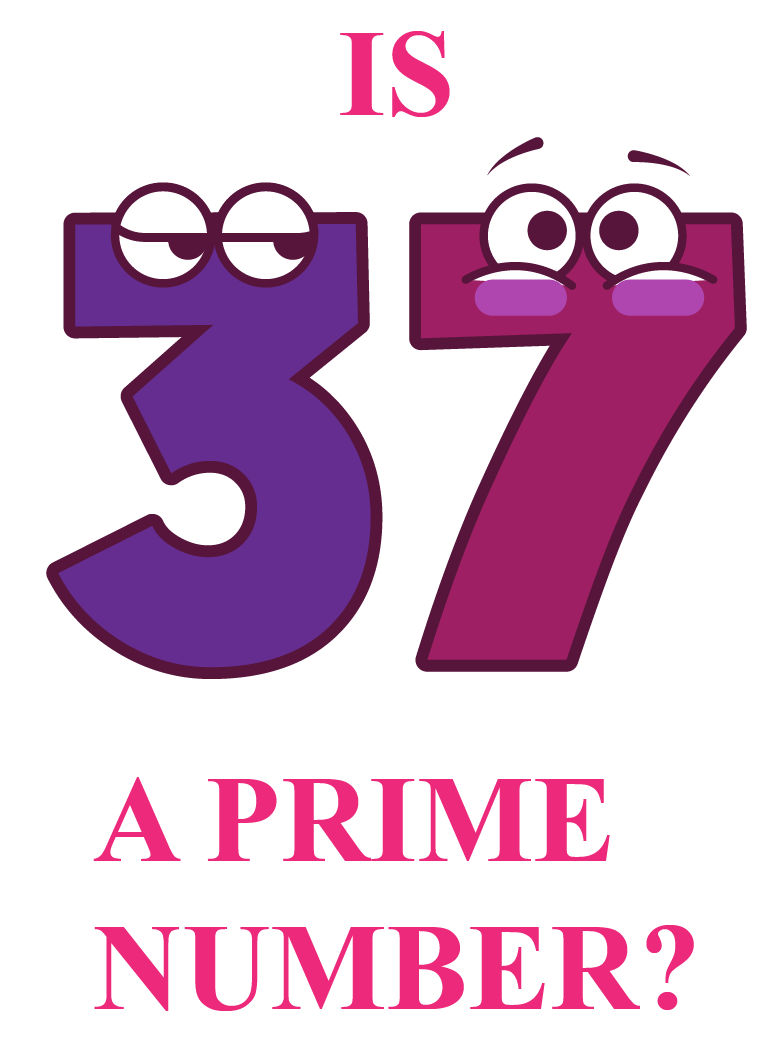Can you divide 37 into equal groups of 2? equal groups of 3 ? groups of 4?
Let us find an answer to this question.

In this mini-lesson, we will explore the world of prime numbers by finding the answers to the questions like what is a prime number, is 37 a prime number, or if  37 is a composite number etc., with help of solved examples and interactive questions.

## Lesson Plan

 1 Is 37 A Prime Number? 2 Solved Examples on Is 37 a Prime Number 3 Important Notes on Is 37 a Prime Number 4 Interactive Questions on Is 37 a Prime Number 5 Challenging Questions on Is 37 a Prime Number

## Is 37 A Prime Number?

Any number, greater than 1, having only two factors, 1 and the number itself is known as a prime number.

To determine if 37 is a prime number, let us see the factors of 37 by the division method.

$$37 \div 1 = 37$$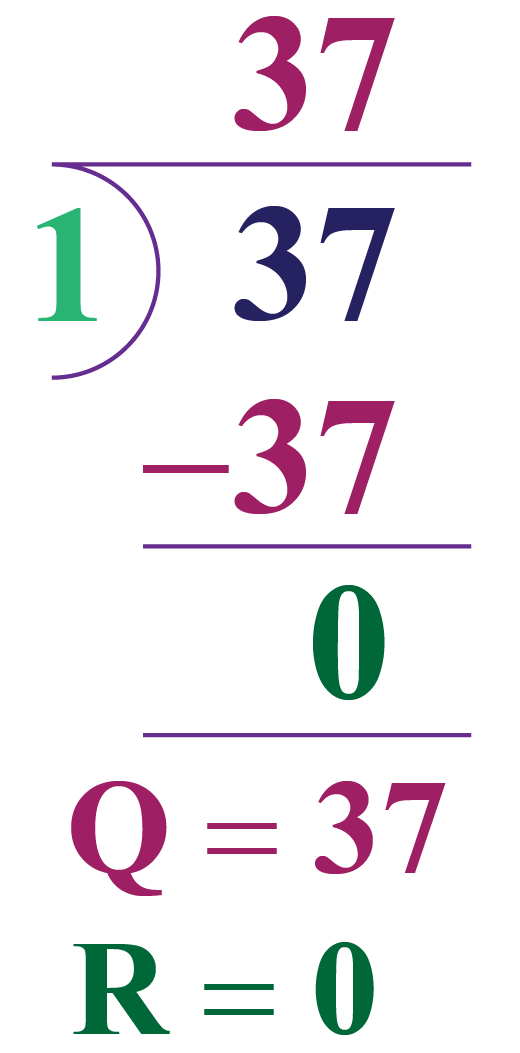Let us divide

$$37 \div 2$$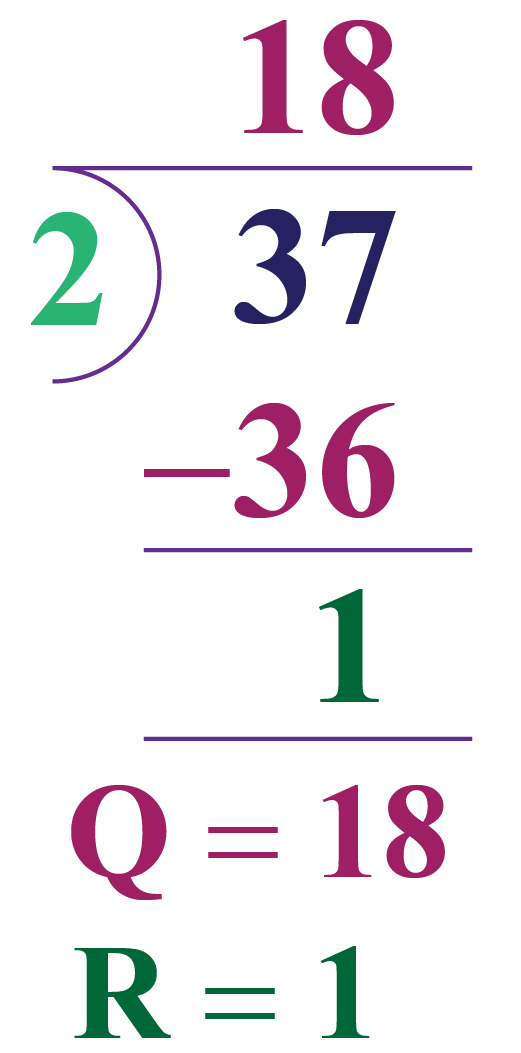$$37 \div 2$$ leaves a remainder similarly any other number will leave a remainder.

We cannot divide 37 with any other natural number as it leaves a remainder and no other number divides 37 completely.

Hence, 37 has only 2 factors 1 and 37. So, 37 is a prime number.

## Is 37 A Composite Number?

A composite number is a natural number that has more than two factors.

37 is not a composite number as it has only two factors, that is, 1 and 37

## Solved Examples

 Example 1

Lucy has to list prime numbers between 37 and 73. Help her list them.

Solution

The prime number chart based on Sieve of Eratosthenes algorithm  is shown below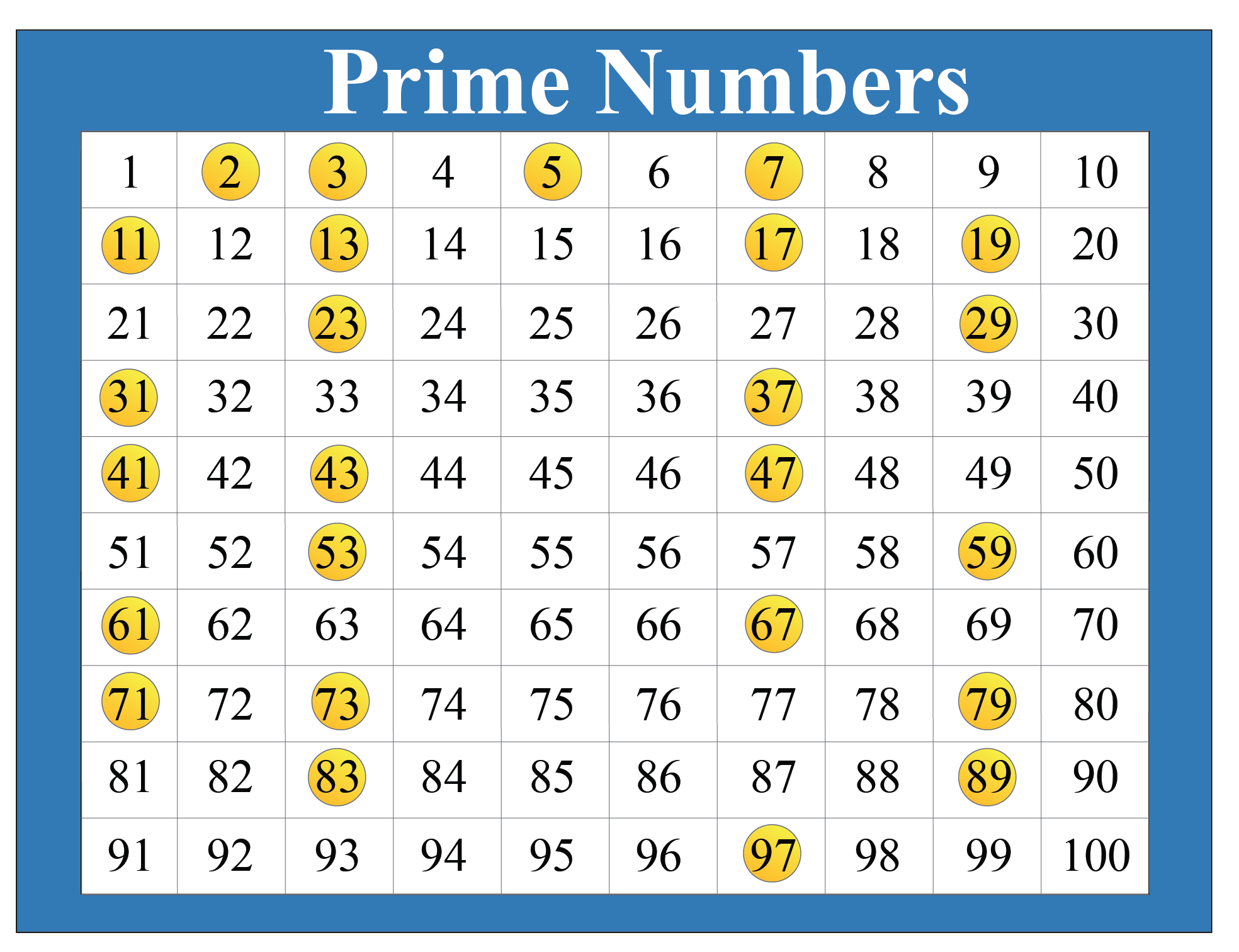From the above list of prime numbers, Lucy listed the following prime numbers between 37 and 73. They are:

41, 43, 47, 53, 59, 61, 67, and 71

She found there were 8 prime numbers between 37 to 73

 $$\therefore$$ Prime numbers between 37 and 73 are 41, 43, 47, 53, 59, 61, 67, and 71
 Example 2

John has to prove that 1 added or subtracted to 37, makes it a composite number. How will he prove it?

Solution

When 1 is added to 37, John will get 38

When 1 is subtracted from 37, he will get 36

36 and 38 are even numbers and they are divisible by 2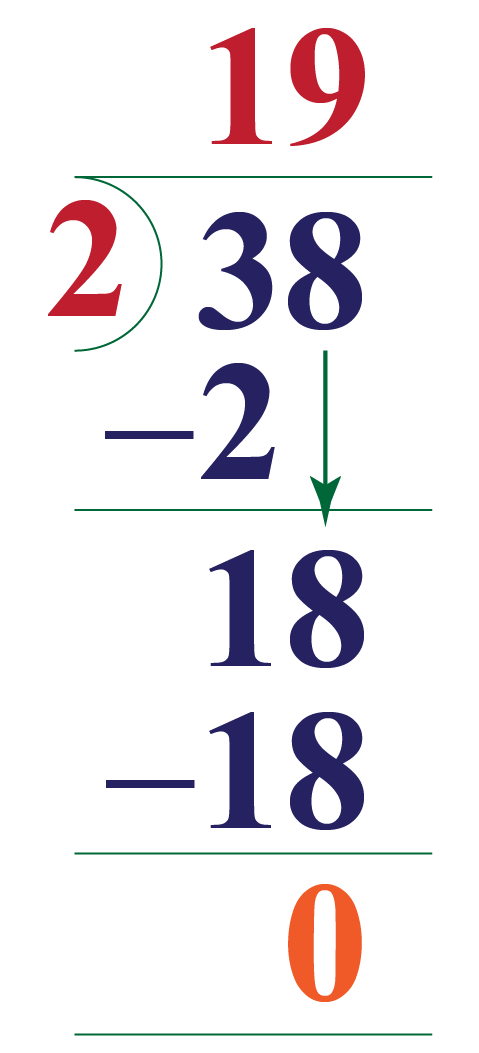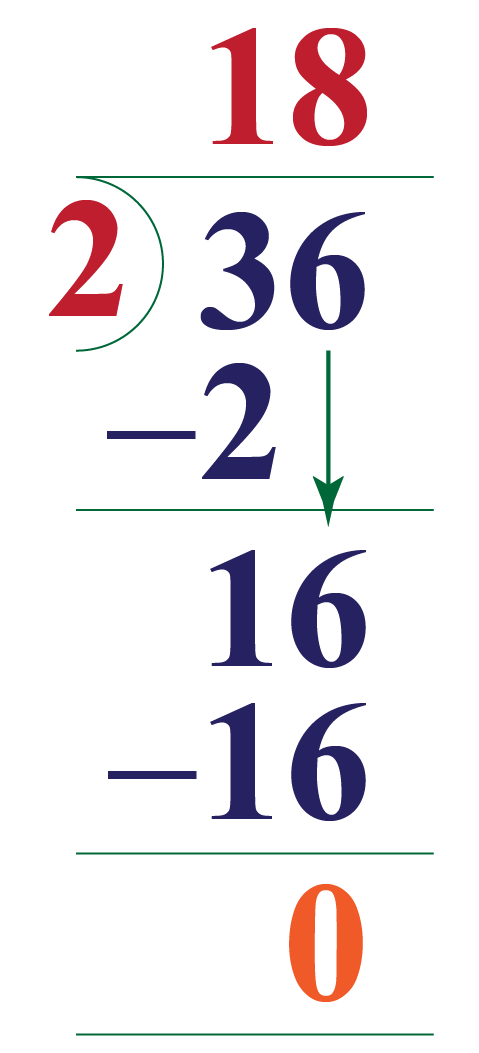Since 36 and 38 are divisible by 2, they have more than 2 factors, and therefore 36 and 38 are not prime numbers.

 $$\therefore$$ 36 and 38 are composite numbers.

More Important Topics
Numbers
Algebra
Geometry
Measurement
Money
Data
Trigonometry
Calculus